Get inspired by the success stories of our students in IIT JAM MS, ISI  MStat, CMI MSc DS.  Learn More

# ISI MStat PSB 2008 Problem 3 | Functional equation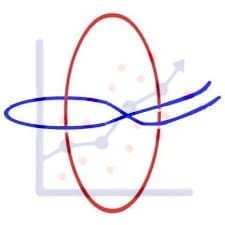This is a very beautiful sample problem from ISI MStat PSB 2008 Problem 3 based on Functional equation . Let's give it a try !!

## Problem- ISI MStat PSB 2008 Problem 3

Let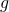be a continuous function with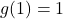such that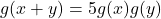for all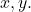Find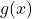.

### Prerequisites

Continuity & Differentiability

Differential equation

Cauchy's functional equation

## Solution :

We are g is continuous function such thatfor all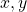and g(1)=1.

Now putting x=y=0 , we get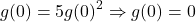or ,.

If g(0)=0 , then g(x)=0 for all x but we are given that g(1)=1 . Hence contradiction .

So,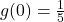.

Now , we can write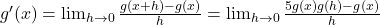(by definition)

Therefore ,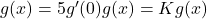, for some constant k ,say.

Now we will solve the differential equation , let y=g(x) then we have from above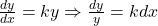. Integrating both sides we get ,c is integrating constant . So , we get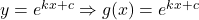Solve the equation g(0)=1/5 and g(1)=1 to get the values of K and c . Finally we will get ,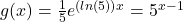.

But there is a little mistake in this solution .

What's the mistake ?

Ans- Here we assume that g is differentiable at x=0 , which may not be true .

Correct Solution comes here!

We are given thatfor allNow taking log both sides we get ,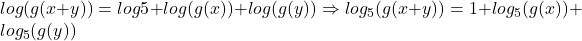, where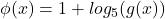It's a cauchy function as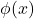is also continuous . Hence ,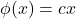, c is a constant.

Now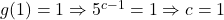.

Therefore ,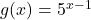## Food For Thought

Letbe a non-constant , 3 times differentiable function . If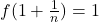for all integer n then find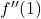.

## Subscribe to Cheenta at Youtube

This is a very beautiful sample problem from ISI MStat PSB 2008 Problem 3 based on Functional equation . Let's give it a try !!

## Problem- ISI MStat PSB 2008 Problem 3

Letbe a continuous function withsuch thatfor allFind.

### Prerequisites

Continuity & Differentiability

Differential equation

Cauchy's functional equation

## Solution :

We are g is continuous function such thatfor alland g(1)=1.

Now putting x=y=0 , we getor ,.

If g(0)=0 , then g(x)=0 for all x but we are given that g(1)=1 . Hence contradiction .

So,.

Now , we can write(by definition)

Therefore ,, for some constant k ,say.

Now we will solve the differential equation , let y=g(x) then we have from above. Integrating both sides we get ,c is integrating constant . So , we getSolve the equation g(0)=1/5 and g(1)=1 to get the values of K and c . Finally we will get ,.

But there is a little mistake in this solution .

What's the mistake ?

Ans- Here we assume that g is differentiable at x=0 , which may not be true .

Correct Solution comes here!

We are given thatfor allNow taking log both sides we get ,, whereIt's a cauchy function asis also continuous . Hence ,, c is a constant.

Now.

Therefore ,## Food For Thought

Letbe a non-constant , 3 times differentiable function . Iffor all integer n then find.

## Subscribe to Cheenta at Youtube

This site uses Akismet to reduce spam. Learn how your comment data is processed.

### One comment on “ISI MStat PSB 2008 Problem 3 | Functional equation”

1.abhishek sinha says:

This solution is incomplete as it assumes that the function is differentiable at zero. Upon taking logarithm of both sides, the functional equation can be converted to a form of Cauchy's functional equation (https://en.wikipedia.org/wiki/Cauchy%27s_functional_equation) and its solution can be inferred from it.

### Knowledge Partner